# Basic Proportionality Theorem

Go back to  'Triangles'

## Introduction:

A famous Greek mathematician Thales gave an important truth relating two equi-angular triangles,i.e., "The ratio of any two corresponding sides in two equiangular triangles is always the same". Thales used a result called the Basic Proportionality Theorem for the same. Before discussing other criterions and theorems of similar triangles, it is important to understand this very fundamental theorem related to triangles: the Basic Proportionality Theorem or BPT Theorem. This theorem is a key to understanding the concept of similarity better.

## Basic Proportionality Theorem:

Basic Proportionality Theorem states that "If a line is drawn parallel to one side of a triangle to intersect the other two sides in distinct points, the other two sides are divided in the same ratio".

In the following figure, segment $$DE$$ is parallel to the side $$BC$$ of $$\Delta ABC$$. Note how $$DE$$ divides $$AB$$ and $$AC$$ in the same ratio: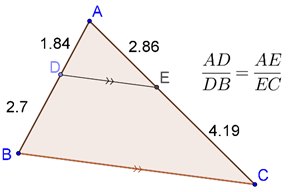## Proof of Basic Proportionality Theorem:

Given:

1. $$\Delta ABC$$
2. $$DE\parallel BC$$

To prove: $$\frac{{AD}}{{DB}} = \frac{{AE}}{{EC}}$$

Construction:

1. Join $$BE$$ and $$CD$$
2. Draw $$DP \bot AC$$
3. Draw $$EQ \bot AB$$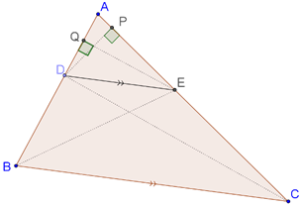Proof: Consider $$\Delta AED$$. If you have to calculate the area of this triangle, you can take $$AD$$ to be the base, and $$EQ$$ to be the altitude, so that:

${\rm{ar}}\left( {\Delta AED} \right) = \frac{1}{2} \times AD \times EQ$

Now, consider $$\Delta DEB$$. To calculate the area of this triangle, you can take $$DB$$ to be the base, and $$EQ$$ (again) to be the altitude (perpendicular from the opposite vertex $$E$$ ).

Thus,

${\rm{ar}}\left( {\Delta DEB} \right) = \frac{1}{2} \times DB \times EQ$

Next, consider the ratio of these two areas you have calculated:

$\frac{{{\rm{ar}}\left( {\Delta AED} \right)}}{{{\rm{ar}}\left( {\Delta DEB} \right)}} = \frac{{\frac{1}{2} \times AD \times EQ}}{{\frac{1}{2} \times DB \times EQ}} = \frac{{AD}}{{DB}}$

In an exactly analogous manner, you can evaluate the ratio of areas of $$\Delta AED$$ and $$\Delta EDC$$:

$\frac{{{\rm{ar}}\left( {\Delta AED} \right)}}{{{\rm{ar}}\left( {\Delta EDC} \right)}} = \frac{{\frac{1}{2} \times AE \times DP}}{{\frac{1}{2} \times EC \times DP}} = \frac{{AE}}{{EC}}$

Finally, We know that "Two triangles on the same base and between the same parallels are equal in area". Here, $$\Delta DEB$$ and $$\Delta EDC$$ are on the same base $$DE$$ and between the same parallels – $$DE\parallel BC$$ .

$\Rightarrow ar\left( {\Delta DEB} \right) = ar\left( {\Delta EDC} \right)$

Considering above results, we can note,

${\frac{{ar\left( {\Delta AED} \right)}}{{ar\left( {\Delta DEB} \right)}} = \frac{{ar\left( {\Delta AED} \right)}}{{ar\left( {\Delta EDC} \right)}}}$

${ \Rightarrow \boxed{\frac{{AD}}{{DB}} = \frac{{AE}}{{EC}}}}$

This completes our proof of the fact that $$DE$$ divides $$AB$$ and $$AC$$ in the same ratio.

Hence Proved.

✍Note: $$DE$$ is parallel to $$BC$$ was crucial to the proof. Without this, the areas of $$\Delta DEB$$ and $$\Delta EDC$$ would not have been the same, and hence the two ratios would have been different.

Can we say that the converse of the Basic Proportionality Theorem (BPT) will hold? That is if, in a triangle, a line segment divides two sides in the same ratio, will it be parallel to the third side? The answer is yes. Let’s prove this result.

## The converse of BPT

The converse of BPT states that "In a triangle, if a line segment intersecting two sides and divides them in the same ratio, then it will be parallel to the third side".

## Proof of converse of BPT:

Consider the following figure,

It is given that $${\frac{{AD}}{{DB}} = \frac{{AE}}{{EC}}}$$ .

Now, Suppose that $$DE$$ is not parallel to $$BC$$ . Draw segment $$DF$$ through $$D$$ which is parallel to $$BC$$, as shown: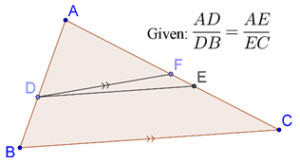Using the BPT, we see that $$DF$$ should divide $$AB$$ and $$AC$$ in the same ratio. Thus, we should have:

$\frac{{AD}}{{DB}} = \frac{{AF}}{{FC}}$

But it is already given to us that:

$\frac{{AD}}{{DB}} = \frac{{AE}}{{EC}}$

This means that:

$\frac{{AE}}{{EC}} = \frac{{AF}}{{FC}}$

Adding 1 to both sides, we have,

\begin{align} \frac{{AE}}{{EC}} + 1 &= \frac{{AF}}{{FC}} + 1 \hfill \\ \Rightarrow \frac{{AE + EC}}{{EC}} &= \frac{{AF + FC}}{{FC}} \hfill \\ \Rightarrow \frac{{AC}}{{EC}} &= \frac{{AC}}{{FC}} \hfill \\ \end{align}

$\Rightarrow \boxed{EC = FC}$

This cannot happen if $$E$$ and $$F$$ are different points, so they must coincide. Thus, we can conclude that $$DE$$ is parallel to $$BC$$, hence completing the proof of the converse of the BPT.

Hence Proved.

## Solved Examples

Example 1: Consider $$\Delta ABC$$, in which segment $$DE$$ parallel to $$BC$$ is drawn from $$D$$ on $$AB$$ to $$E$$ on $$AC$$, as shown below: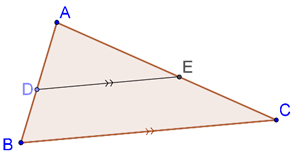Show that $$\Delta ADE$$ is similar to $$\Delta ABC$$.

Solution: Recall that two triangles are said to be similar if they are equi-angular (corresponding angles are equal), and corresponding sides are proportional. It is obvious that $$\Delta ADE$$ and $$\Delta ABC$$ are equi-angular, since:

1. $$\angle A$$ = $$\angle A$$ (common angle)

2. $$\angle ADE$$ = $$\angle ABC$$ (corresponding angles)

3. $$\angle AED$$ = $$\angle ACB$$ (corresponding angles)

Now, we will show that the corresponding sides are proportional. Using the BPT, we have:

\begin{align}\frac{{AD}}{{DB}} &= \frac{{AE}}{{EC}}\\ \Rightarrow \quad \frac{{DB}}{{AD}} &= \frac{{EC}}{{AE}}\\ \Rightarrow \quad \frac{{DB}}{{AD}} + 1 &= \frac{{EC}}{{AE}} + 1\\ \Rightarrow \quad \frac{{DB + AD}}{{AD}} &= \frac{{EC + AE}}{{AE}}\\ \Rightarrow \quad \frac{{AB}}{{AD}} &= \frac{{AC}}{{AE}}\\ \Rightarrow \quad \frac{{AD}}{{AB}} &= \frac{{AE}}{{AC}}\end{align}

We have thus shown two pairs of sides to be proportional. All that remains to be shown that the ratios above are also equal to the ratio of the third pair, that is, to $$\frac{{DE}}{{BC}}$$.

To prove that, draw segment $$EF$$ parallel to $$AB$$, as shown: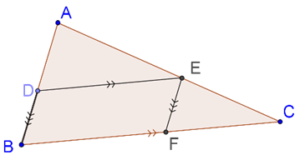Since $$EF\parallel AB$$, the BPT tells us that:

\begin{align}\frac{{CE}}{{EA}} &= \frac{{CF}}{{FB}}\\ \Rightarrow \quad \frac{{CE}}{{EA}} + 1 &= \frac{{CF}}{{FB}} + 1\\ \Rightarrow \quad \frac{{CE + EA}}{{EA}} &= \frac{{CF + FB}}{{FB}}\\ \Rightarrow \quad \frac{{AC}}{{AE}} &= \frac{{BC}}{{FB}}\end{align}

But $$BF$$ is equal to $$DE$$ (since $$DEFB$$ is a parallelogram), and so:

\begin{align}\frac{{AC}}{{AE}} &= \frac{{BC}}{{DE}}\\ \Rightarrow \quad \frac{{AE}}{{AC}} &= \frac{{DE}}{{BC}}\end{align}

Thus, we finally have:

$\frac{{AD}}{{DB}} = \frac{{AE}}{{AC}} = \frac{{DE}}{{BC}}$

This proves that all the three pairs of sides are proportional, which means that the two triangles are similar.

$\Rightarrow \boxed{\Delta ADE \sim \Delta ABC}$

✍Note: The result we have discussed here is extremely important and will be used frequently, so it is imperative that you go through the discussion again if you have not followed it completely.Challenge: $$E$$ and $$F$$ are points on the sides $$PQ$$ and $$PR$$ respectively of a $$\Delta PQR$$ . For the following case, state whether $$EF\parallel QR$$ ,

$$PQ = 1.28{\text{ cm}}$$, $$PR = 2.56{\text{ cm}}$$, $$PE = 0.18{\text{ cm}}$$ and $$PF = 0.36{\text{ cm}}$$

⚡Tip: Apply converse of BPT.

Example 2: $$ABCD$$ is a trapezium such that $$AB\parallel DC$$ . $$E$$ and $$F$$ are points on non-parallel sides $$AD$$ and $$BC$$ respectively such that $$EF\parallel AB$$, as shown below: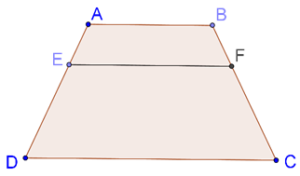Show that $$\frac{{AE}}{{ED}} = \frac{{BF}}{{FC}}$$ .

Solution: Join $$BD$$, and let it intersect $$EF$$ at $$G$$, as shown below: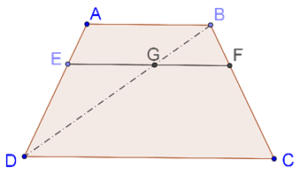Now, we will apply the BPT twice, once in $$\Delta ADB$$, and once in $$\Delta DBC$$. We have:

\left\{ \begin{align}&\frac{{AE}}{{ED}} = \frac{{BG}}{{GD}}\\&\frac{{BG}}{{GD}} = \frac{{BF}}{{FC}}\end{align} \right.

Clearly,

$\boxed{\frac{{AE}}{{ED}} = \frac{{BF}}{{FC}}}$Challenge: $$ABCD$$ is a trapezium in which $$AB\parallel DC$$ and its diagonals intersect each other at the point $$O$$ . Show that $$\frac{{AO}}{{BO}} = \frac{{CO}}{{DO}}$$ .

⚡Tip: Draw $$EO$$ sucht that $$E$$ is a point on $$AD$$ and $$EO\parallel AB$$ , then apply BPT in $$\Delta DAB$$ and $$\Delta ADC$$ .

Example 3: Consider the following figure: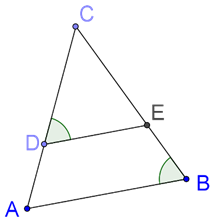It is given that $$\frac{{CD}}{{DA}} = \frac{{CE}}{{EB}}$$, and $$\angle CDE$$ = $$\angle CBA$$. Prove that $$\Delta CAB$$ is isosceles.

Solution: We are given that $$\frac{{CD}}{{DA}} = \frac{{CE}}{{EB}}$$

So by the converse of the BPT, we can note,

$DE\parallel AB$

Thus,

$\angle CDE = \angle CAB{\text{ }}\left( {{\text{corresponding angles}}} \right)$

But it is also given that,

$\angle CDE = \angle CBA$

Which means that,

$\angle CAB = \angle CBA$

$\Rightarrow \boxed{CA = CB}$

Hence $$\Delta CAB$$ is isosceles.Challenge: Using the Basic Proportionality Theorem, prove that a line drawn through the mid-point of one side of a triangle parallel to another side bisects the third side.

⚡Tip: Since the line is drawn through the mid-point, so it is dividing the side of the triangle in equal proportion and this theorem is also known as mid-point theorem.